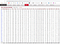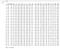# Another Baseball Simulator… in Python!

Two weeks ago, I did a little bit of web scraping, getting data from a simple table. Last week, I created a baseball simulator in JavaScript. This week, I’m combining the two: scraping data from a baseball stats site, then building a simulator… but this time, both in Python!

## Scraping The Data

Whereas in JavaScript we used the request().then().then() function syntax along with the DOM querySelector() and querySelectorAll() functions to find the table, we’ll be using the Python equivalents for this exercise: the ‘requests’ library for the initial GET request and then the famous ‘Beautiful Soup’ library to filter through the web page and get the desired table.

After downloading said libraries locally on your machine (via pip, conda or whatever your preference is), import the functions at the top of your file. Then, to get the data from the website, we use requests.get() and then several BeautifulSoup functions to parse the data:

`import requestsfrom bs4 import BeautifulSoupimport pandas# Gets all the HTML from the given URLpage = requests.get("https://www.baseball-reference.com/leagues/MLB/2019-standard-batting.shtml")# Parses the HTML string and turns it into a analyzable Beautiful Soup objectsoup = BeautifulSoup(page.content, 'html.parser')# Getting the first table from the pagemy_table = soup.find('table')# Getting the column header cells, which contains column labelsmy_head = my_table.find('thead')# Getting the inner text from each cell - like .innerText in JSmy_head = [cell.text for cell in my_head.find_all('th')]# All the rows containing team batting totals - AB, R, H, etc.my_table = [row for row in my_table.find_all('tr')]# Getting the inner text from each row cell, i.e. the numbers, and converting them from strings to floatsmy_table = [[float(cell.text) for cell in row.find_all('td')] for row in my_table]# Filters out the empty cells my_table = [cell for cell in my_cells if cell]`The table we’re scraping- contains total batting data for every team in the MLB!

At this point, we invoke our last library: the magical Pandas, with its core DataFrame data structure, to hold our batting #s as a sort of Excel-style spreadsheet, which can be easily manipulated and filtered:

`# Taking our arrays of arrays of numbers (my_table) and inserting them into a DataFrame to make a 2-D data table; the array of labels in my_head will serve as the columnstats = pandas.DataFrame(data=my_table, columns=my_head[1:])# Filtering out the last row, which contains unneeded sums & averages of the individual 30 team rowsstats = stats.iloc[:30, :]`

Using the wonderful Jupyter Notebook tool, which lets us run and tests individual code snippets, here’s what we get:A Pythonic version of the Baseball Reference table from our initial page

Even though these rows are team-wide averages, we can treat them as individual player stats and later load them into our simulator. We don’t need all 28 columns; so we can just grab the key columns from this table and use them to generate a probability distribution for each “player”. Then, using our knowledge of baseball, we can calculate the % of plate appearances resulting in singles, doubles, triples, homers, walks and outs:

`# Paring our stats DataFrame from 28 columns to 10df = stats[['PA', 'H', '2B', '3B', 'HR', 'BB', 'GDP', 'HBP', 'SH', 'SF']]# Calculating the number of singles (i.e. hits - non-single hits)df['1B'] = df['H'] - df[['2B', '3B', 'HR']].sum(1)# Adding together total walks: 4-ball walks + hit by pitch walksdf['WALK'] = df[['BB', 'HBP']].sum(1)# Outs = All plate appearancesdf['OUT'] = df['PA'] - df[['H', 'WALK']].sum(1)# The needed columns for our 6 outcomesdf_probs = df[['1B', '2B', '3B', 'HR', 'WALK', 'OUT']]# Dividing the rows by total number of plate appearances, to  get probabilities that add up to 1 for each rowdf_probs = df_probs.div(df_probs.sum(1), axis=0)`

Here’s what our probability distribution looks like now:

Now, we can treat these 30 rows as players and load them onto teams! We’ll randomly select two groups of 9 rows and treat them as 9-player teams:

`team1 = df_probs.sample(9)avails = [ix for ix in df_probs.index if ix not in team1.index]team2 = df_probs.iloc[avails, :].sample(9)`

Now, let’s go through and create the game & simulator classes! We can basically just take the code from our JavaScript classes and convert them into Python, with several alterations to take into account the use of DataFrames and player-specific data:

`class Player:    def __init__(self, probs):        self.probs = pd.Series(probs) # Player prob distribution        self.stats = [] # Player at-bat results will be stored here            # Randomly select number from 0 to 1; probability of outcomes will depend on individual player probs. Then, store in player stats    def at_bat(self):        outcome = np.random.choice(self.probs.index, p=self.probs.values)        self.stats.append(outcome)        return outcome    # Calculate's player on-base percentage    def OBP(self):        nonouts = [ab for ab in self.stats if ab != 'OUT']        return 1.0 * len(nonouts) / len(self.stats)        # Calculates player batting average    def AVE(self):        apps = [ab for ab in self.stats if ab != 'WALK']        hits = [ab for ab in apps if ab != 'OUT']        return 1.0 * len(hits) / len(apps)        # Records number of bases for each outcome (e.g. single = 1, double = 2)    def bases(self, hit_type):        if hit_type in ['WALK', '1B']:            return 1        elif hit_type == '2B':            return 2        elif hit_type == '3B':            return 3        elif hit_type == 'HR':            return 4        else:            return 0        # Slugging = average number of bases advanced per at-bat (counting walks as 1 base, slightly different from standard definition)      def slugging(self):        return sum([self.bases(ab) for ab in self.stats]) / len(self.stats)`

Next, we create our team class, which is initialized with a 9-row DataFrame, each row serving as a player’s probability distribution:

`class Team:    def __init__(self, players):        self.players=players # 9x6 DataFrame        self.record = [0, 0] # Initial 0-0 record, updated after each game    # Adds one to win or loss column    def update_record(self, boo):        if boo:            self.record += 1        else:            self.record += 1`

Now we create the Game class, which contains the functions for generating each at-bat and updating the game state accordingly:

`class Game:    def __init__(self,                 teams,                 inning=1,                 outs=0,                 away_or_home=0,                 bases=[0,0,0],                 score=[0,0],current_player=[0,0]):        self.teams=teams        self.inning=inning        self.outs=outs        self.away_or_home=away_or_home        self.bases=bases        self.score=score        self.game_on=True        self.current_player=current_playerdef walker(self):        self.bases.append(0)        self.bases += 1        for i in range(3):            if self.bases[i]==2:                self.bases[i] -= 1                self.bases[i+1] += 1        runs = self.bases[-1]        self.bases = self.bases[:3]        self.score[self.away_or_home] += runsdef hitter(self, hit_type):        if hit_type == '1B':            self.bases = [1,0]+self.bases        elif hit_type == '2B':            self.bases = [0,1]+self.bases        elif hit_type == '3B':            self.bases = [0,0,1]+self.bases        elif hit_type == 'HR':            self.bases = [0,0,0,1]+self.bases        runs = sum(self.bases[3:])        self.bases = self.bases[:3]        self.score[self.away_or_home] += runsdef handle_at_bat(self):        player=self.teams[self.away_or_home].players[self.current_player[self.away_or_home]]        result = player.at_bat()        if result == 'OUT':            self.outs += 1        elif result == 'BB':            self.walker()        else:            self.hitter(result)        if (self.inning >= 9 and ((self.outs >= 3 and self.away_or_home == 0) or self.away_or_home == 1) and self.score < self.score) or (self.inning >= 9 and self.outs >= 3 and self.score > self.score):            self.game_on = False        if self.outs >= 3:            if self.away_or_home == 1:                self.inning += 1            self.outs = 0            self.current_player[self.away_or_home] = (self.current_player[self.away_or_home] + 1) % 9            self.away_or_home = (self.away_or_home + 1) % 2            self.bases = [0, 0, 0]def play_game(self):        while self.game_on:            self.handle_at_bat()        final_score = copy.copy(self.score)        winner = 1 if (self.score < self.score) else 0        self.teams.record[winner] += 1        self.teams.record[(winner+1)%2] += 1        self.inning = 1        self.outs = 0        self.away_or_home = 0        self.bases = [0,0,0]        self.score = [0,0]        self.game_on = True        return {            "final_score": final_score,            "winner": winner        }`

Lastly, we add the Simulator class, which takes the inputs specified by the player (game state, players) and simulates a given number of games, as determined by the user:

`class Simulator:    def __init__(self, teams, inning=1, away_or_home=0,bases=[0,0,0], outs=0, score=[0,0]):        self.teams=teams        self.inning=1        self.outs=0        self.away_or_home=away_or_home        self.bases=[0,0,0]        self.score=[0,0]        def simulate(self, its=100):        game_log = []        wins = 0        for i in range(its):            game = Game([getattr(self, attr) for attr in dir(g) if "__" not in attr])            result = game.play_game()            wins += result.winner            game_log.append(result)        print(f"The home team won \${wins} out of \${its}, for a winning percentage of {wins / its * 100}%!")        return game_log`